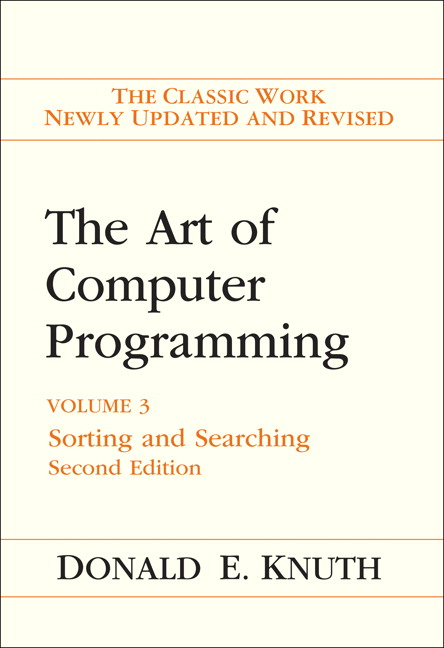Lab Week 11: Sorting

Overview

1. Sorting
2. Divide & Conquer Strategies
3. Parallel Sorting
4. Lab 05: Sorting
5. Sorting Networks

Sorting

Sorting is a fundamental operation in computer scienceGoal

[5, 7, 2, 3, 5, 2, 8, 1, 1, 5]

and transform it into a sorted array

[1, 1, 2, 2, 3, 5, 5, 5, 7, 8]

Same elements in increasing order.

Simplistic Sorting Strategies

Selection Sort

1. Find smallest element; put at index 1
2. Find next smallest; put at index 2
5                        7                        2                        3

Insertion sort

1. Iterate j = 1, 2, ...
2. Insert jth element in sorted order by pairwise swaps
5                        7                        2                        3

Bubble sort

• Repeat until sorted:
1. Iterate over array:
2. Swap adjacent pairs if out of order
5                        7                        2                        3

Questions

• Are any of these strategies efficient?
• Can they be parallelized easily?
• Are these algorithms practical?

Faster Sequential Algorithms: Divide and Conquer

Merge Sort

1. Divide array in half
2. Sort left half (recursively)
3. Sort right half (recursively)
4. Merge sorted halves
7                        5                        3                        2

Randomized Quick Sort

1. Pick random “pivot” element
2. Put all smaller elements on left
3. Put all larger elements on right
4. Recursively sort left/right sides
5           4            7            9            2            8           3

Questions

• Are any of these strategies efficient?
• Can they be parallelized easily?
• Are these algorithms practical?

Quicksort in More Depth

1. If we’re unlucky, it can be slow
• e.g., always pick smallest/largest element as pivot
2. In practice it tends to be fast
• it is extremely unlikely that we are often unlucky
3. Many built-in sorting procedures are variants of quicksort

Parallelizing Quicksort

Sequential:

• Select pivot
• Divide array
• left half smaller than pivot
• right half larger than pivot

Parallel:

• Sort left half
• Sort right half

Question

Why is parallelization potentially problematic?

Overcoming Imbalance

Efficient, as long as no idle processes

• Thread pools are good for this!
• Need to ensure tasks performed in correct order

Implementation

Recall Fork-Join pools:

• thread pool with efficient support for forking:
• combine solutions (if necessary)

Creating FJ pool:

import java.util.concurrent.ForkJoinPool;
...
ForkJoinPool pool = new ForkJoinPool(POOL_SIZE);
...

Recursive Actions

Tasks for fork-join pools (without return values)

• Extend RecursiveAction
• Override compute() method
...
@Override
protected void compute () {

//... compute stuff ...//

sub1.join();                    // wait for sub1 to complete
sub2.join();                    // wait for sub2 to complete

//... compute more stuff stuff ...//
}
}

Parallel Implementation of Quicksort

Basic task: Sort array between index i and j

Lab 05: Sorting (Optional)

• Write a method that sorts a large array of doubles as quickly as possible
• large = > 1 million elements
• Should be faster than Arrays.sort()

Suggestions

• Quicksort is a good starting point
• Use ForkJoinPool
• Use a reasonably large base case
• Arrays.sort() as a sub-routine
• it is quite fast for smaller arrays!
• Be careful about memory access pattern
• cache performance is crucial for large arrays

Sorting Networks

Insertion Sort, Revisited

for (int i = 1; i < data.length; ++i) {
for (int j = i; j > 0; --j) {
if (data[j-1] > data[j]) {
swap(data, j-1, j)
}
}
}

Comparators: Visualizing Swaps

if (data[i] > data[j]) {
swap(data, i, j)
}

Insertion Sort, Visualized

for (int i = 1; i < data.length; ++i) {
for (int j = i; j > 0; --j) {
if (data[j-1] > data[j]) {
swap(data, j-1, j)
}
}
}

Measuring Speed

depth = max # of comparators on any path from input to output

Bubble Sort, Revisited

for (int m = data.length - 1; m > 0; --m) {
for (int i = 0; i < m; ++i) {
if (data[i] > data[i+1]) {
swap(data, i, i+1)
}
}
}

Huh

• Insertion sort and bubble sort perform precisely same operations
• only differ in the order in which comparisons are made
• When fuly parallelized, both are same sorting network

• Parallel versions are reasonably efficient
• depth $\approx 2 n$

Current State

What is known:

• Optimal depth sorting networks for $n \leq 17$

What is not known:

• Optimal depth sorting networks for $n \geq 18$